#### Encyclopedia of Microtonal Music Theory

Private lessons with Joseph Monzo are available online via Discord / WhatsApp / Skype: composition, music-theory, tuning-theory, piano, and all woodwinds (sax, clarinet, flute, bassoon, recorder). Current rates US\$ 80 per hour (negotiable). Send an email to: monzojoe (AT) gmail.

# on Harmonic Entropy

by Paul Erlich, with commentary by Joe Monzo

based on postings to the Mills College Tuning Digest

original text indented

commentary by Joe Monzo based on discussion with the author

Original text available here

Erlich is here providing a nice mathematical framework to support the common observation that simpler ratios are important musical perceptual gestalts.

From: Paul Erlich
To: Tuning Digest

[posted to the Tuning Digest since September of 1997]

I do believe that the place and periodicity mechanisms are both at play.

The place and periodicity mechanisms are the two different ways we apparently perceive pitch.

Place seems to work for the whole spectrum, but more accurately for high notes. It is a result of the stimulation of hairs along the basilar membrane in the cochlea of the inner ear, which are connected to and stimulate auditory nerves, in response to the vibration of air molecules. The pitch we perceive is directly related to the location along the length of the membrane at which the hairs are stimulated, thus Erlich is calling it a "place" mechanism.

Periodicity seems to work only for low notes (< approximately 1 kHz). It is a result of some kind of mathematical analysis that is going on in the brain whereby we recognize patterns of nerve stimulation. It is unclear as yet exactly how this works. Erlich's Harmonic Entropy is one model of it.

I also believe that Plomp's model gives a fine account of the place-related component of dissonance, which I like to call roughness. Combination tones complicate the matter but with a knowledge of the amplitudes and frequencies of all combination tone components, Plomp's algorithm can be still applied.

The basis for Plomp's (and Sethares's) theories: when the critical bands for two frequencies overlap they create roughness.

This is close to Helmholtz's ideas, but his "beating" is not as important as roughness. Beating can be in the audible frequency range, but it won't create a pitch because it is just a variation in volume at a certain frequency.

The Sethares theory concerns the role of the place mechanism in sonance. Erlich's Harmonic Entropy concerns the role of the periodicity mechanism.

But a phenomenon called "virtual pitch" or "fundamental tracking" is central to Parncutt's treatment of dissonance and does represent, I believe, an additional factor besides critical band roughness. This phenomenon is clearly distinct from the combination tone phenomenon, but it may have a lot to do with periodicity mechanisms. There is a very strong propensity for the ear to try to fit what it hears into one or a small number of harmonic series, and the fundamentals of these series, even if not physically present, are either heard outright, or provide a more subtle sense of overall pitch known to musicians as the "root". As a component of consonance, the ease with which the ear/brain system can resolve the fundamental is known as "tonalness." I have proposed a concept called "relative harmonic entropy" to model this component of dissonance.

Sonance has 2 components: tonalness (Parncutt/Erlich) and roughness (Plomp/Sethares).

With dyads of harmonic timbres, both components give similar sonance rankings; i.e., a 3/2 will have higher tonalness and lower roughness than a 15/11.

For sine waves, simple integer ratios no longer minimize roughness, but they still maximize tonalness.

For inharmonic timbres, Sethares can find a whole new set of intervals that have low roughness (= are consonant), but they don't have high tonalness, because they don't imply a fundamental.

For triads or higher-ads - the roughness of Otonal chords is the same as that of Utonal chords because they have the same intervals, but the tonalness of Otonal chords is much greater, because they imply a much simpler set of harmonics over a fundamental.

Erlich thinks tonalness has to do with all the subsets of the chord.

The harmonic entropy is based on the concept that the critical band represents a certain degree of uncertainty in the perception of pitch,

this phrase should read:

The harmonic entropy is based on the concept that there is a degree of uncertainty in the perception of pitch,

(Erlich says the original phrase is a naive speculation on the possible connection with the critical band model.)

i.e., 301/200 will be heard as a mistuned 3/2.

and for any "true" interval, the auditory system will perceive a range of intervals spanning a number of simple-integer ratios.

Simple-integer ratios come into the picture because if the heard tones are to be understood as harmonic overtones of some missing fundamental or root, they must form a simple-integer ratio with one another. The range is a sort of probability distribution, and a certain amount of probability is associated with each of the simple-integer ratios.

According to van Eck: the probability distribution of the perceived interval is a bell curve - high when close to the interval and lower the farther away you get.

One way of modeling this is with a Farey series and its mediants. The Farey series of order n is simply the set [of] all the ratios of numbers not exceeding n,

The Farey series gives a list of all the ratios in the harmonic series up to a certain point; i.e., Farey series of order 5 = all ratios of numbers 5 and less

```	0/1 smallest ratio
1/5+
1/4
1/3
2/5
1/2
3/5
2/3
3/4
4/5
1/1
5/4
4/3
3/2
5/3
2/1
5/2
3/1
4/1
5/1
1/0 largest ratio
```
and the mediant between two consecutive fractions in a Farey series is the sum of the numerators over the sum of the denominators (this definition has many mathematical and acoustical justifications).

Mediant = the simplest ratio between any two consecutive ratios in the Farey Series.

A model that works for Erlich is one that conceptualizes a Farey series in the brain that recognizes ratios up to a fairly high point (= a high-order Farey series).

Mediants define the conceptual boundaries of the ratios and since simpler ratios are farther away from more complex ones and complex ones cluster together, there are more possible actual intervals (rational or otherwise) that can be conceived as simpler ones than for more complex ones.

Starting with the perceived interval, the mediant spacing and the bell curve give us a set of probablities of hearing the conceived intervals.

The simpler-integer ratios take up a lot of room, defined as the interval between the mediant below and the mediant above, in interval space, and so are associated with large "slices" of the probability distribution, while the more complex ratios are more crowded and therefore are associated with smaller "slices."

Now the harmonic entropy is defined, just like in information theory, as the sum over all ratios of a certain function of the probability associated with that ratio. The function is x*log(x). (See an information theory text to find out why.) When the true interval is near a simple-integer ratio, there will be one large probability and many much smaller ones. When the true interval is far from any simple-integer ratios, many more complex ratios will all have roughly equal probabilities. The entropy function will come out quite small in the former case, and quite large in the latter case.

[Some examples:]

In the case of 700 cents, 3/2 will have far more probabilty than any other ratio, and the harmonic entropy is nearly minimal.

In the case of 300 cents, 6/5 will have the largest probability in most cases, but 7/6, 13/11, and 19/16 will all have non-negligible amounts of probability, so the harmonic entropy is moderate.

In the case of 100 cents, 15/14, 16/15, 17/16, 18/17, 19/18, 20/19, and 1/1 will all have significant probability, and the harmonic entropy is nearly maximal.

According to Erlich, harmonic entropy can be calculated from those probablities.

In terms of the periodicity model, we can imagine a process which samples the signal for random periods of time (with some probability distribution that is large for very short times and vanishes for long enough times) and in each period, counts the cycles of each pitch to come up with a ratio (or equivalently, to come up with a fundamental frequency, of which the heard note will be harmonic overtones and therefore possess a small-integer ratio by implication). Note that harmonic partials within the heard tones are irrelevant because the cycles here need not be sinusoidal for the counting to occur.

Also, there is some evidence that the ear-brain system adjusts its sampling rate according to the rate of harmonic change in the music.

Harmonic entropy can be measured in bits if 2 is used as the base in the logarithm of the formula.

If logs to the base 2 are used in the definition above, the entropy measures the expected amount of information, in bits, needed in an optimal code to communicate the ratio being heard. So the entropy really measures, in a sense, "cognitive dissonance." Now the exact probability distribution of sampling times, or the order of Farey series one should use, is something that may be difficult to determine. However, as the order of the Farey series is increased more and more, the entropy curve (defined as a function of interval width) continues rising but stops changing shape (I have observed this numerically but not proved it mathematically). In the limit of a Farey series of order infinity, one should find a smooth "relative entropy" curve that gives a good approximation of the ups and downs of the entropy curve for any reasonably large finite order.

In other words, re: Farey series of order infinity - even if we could conceive of intervals as accurately as we want, we would still substitute simpler ratios for more complex ones, because of our perceptual limitations (= the bell curve doesn't change, no matter how high the order), and the fact that simpler ratios always take up more room than more complex ones, no matter how high the order in the Farey series

These curves look remarkably like many of the Helmholtz/Plomp curves that were derived from completely different assumptions, and though they are meant to represent a completely different component of dissonance, they lead to the same conclusions for intervals of tones with some appropriate overtone structure.

This sentence is the link to the speculation noted above regarding the critical band.

However, when three or more notes are involved, the two components of dissonance can have quite different behavior. Consider Partch's "otonal" and "utonal" chords. Adding higher identities to both chords increases the roughness of both by the same amount. But while the periodicity of the otonal chords will be unchanged or perhaps multiplied by small powers of two, the periodicity of the utonal chords increases dramatically. Thus the process of counting will not be significantly complicated, and may even be aided, by adding higher identities to the otonal chords, while in the utonal case the likelihood of counting the same relative numbers of cycles in each sampling period becomes very small, and thus the entropy becomes very large. So the high-limit utonal chords, though just as much minima of roughness as the corresponding otonal chords, are almost impossible to assign a fundamental frequency to and are therefore not minima of harmonic entropy.

With 3-note chords, the bottom line of the graph would become a plane with the 4:5:6 triad consuming a lot of space because of its simple intervals.

Even though 1/4:1/5:1/6 is composed of the same intervals, in terms of periodicity it must be analyzed as 10:12:15 (because the perceived fundamental always is derived from and implies an otonal conception).

Thus, the utonal triad has rapidly ever-increasing periodicity as higher identities are added.

It is often possible for the brain to look for periodicities among some components of the signal and dismiss the rest as "noise." This is why the root of a major triad does not appear to change when the third is decreased from 5/4 through 11/9 to 6/5 and the chord becomes a minor triad; although the minor triad can be understood as 10:12:15, these numbers are already too high for the entropy of the entire signal to be low enough to compete with the low entropy of the perfect fifth alone (10:15 = 2:3); even the major third alone (12:15 = 4:5) is stronger and can dominate if the "third" is in the bass.

In the experiment of changing the 3rd of a major triad from 5/4 > 11/9 > 6/5 the harmonic entropy of 3:2 is so low that the brain will latch onto that and get a strong sense of the root from it.

The ratio 5:4 has a low enough entropy that when it appears in the bass of a minor triad (12:15:20) is gives a sense of "12" as the root of a "major 6th" chord.

The rule is that any subset of higher overtones will imply the same fundamental (because they are all octave-equivalent to it).

In the otonal case, looking at any subset of the notes present (except 9:3, etc.) will lead to a periodicity which is octave-equivalent to, if not identical to, that of the entire chord, so various combinations of components of the signal effect a reinforcement of the tonalness of the overall chord.

The exception (9:3 etc) simply covers ratios which are not in lowest terms i.e., the implied root is 3, because 9:3 = 3:1, so the 3 functions here as 1

Both the rule and the exception can be taken into account by saying that if a number of samples of different subsets are taken, all of the fundamentals they imply will be members of the set of harmonics (partials) of the lowest fundamental (i.e., not just the set of powers of 2), thus they will all imply the same low fundamental if taken together.

How to weigh the various subsets' contributions to the probabilities of particular fundamentals in an overall analysis is unclear. Even without the consideration of subsets, there appears to be no mathematical theory of ratios of three of more numbers analogous to Farey theory, and no easy way to create one. Unlike roughness, tonalness is not merely concerned with pairwise interactions of tones but three-way and higher interactions as well. A mathematical model for it is out of my grasp at the moment.

I think it is fair to say that Harry Partch's Genesis of a Music, for all its inconsistencies, forms a common grounding for a great many of us on this list in our discussions. By the way, I intend to model Partch's "one-footed bride" with a sort of octave-equivalent harmonic entropy function; that is, rather than using a Farey series (or a series such as used by Mann where the sum of numerator and denominator does not exceed a certain limit), using instead the ratios up to a given Partch limit ("odd limit", that is, the largest odd factor of either the numerator or denominator does not exceed a certain limit). Instead of letting the order of the Farey series approach infinity, I will let the odd limit approach infinity, and I expect that for some realistic assumption about pitch resolution, the one-footed bride will emerge.

In discussion with Erlich, it was evident to me also that the graph of harmonic entropy would resemble the "one-footed bride".

When I worked out a model for harmonic entropy, which should also describe critical band roughness if the partials decrease in amplitude in some specific fashion, I derived that to a good approximation, the complexity of a just ratio is directly related to its DENOMINATOR.

DENOMINATOR should read "the smaller term in the ratio", because in some cases the numerater can be the smaller term.

Later, imposing octave equivalence made me change this to ODD LIMIT, but I admit that it's possible that octave equivalence does not really come in to the "objective" dissonance of an interval.

From a later post:

A while back I posted on my concept of harmonic entropy. In February 1997 I ran a computer program to compute the harmonic entropy of all intervals within the octave in 1-cent increments, based on the assumption that our brain can ideally recognize ratios with numerator up to N but our hearing of frequencies is blurred in the form of a normal distribution with standard deviation 1% (based on Goldstein's work). I hadn't looked at the results yet, so as a preliminary study I listed the local minima and maxima below.

Provided you start with a high enough N in the first place, the minima are going to remain more or less the same no matter how accurate you make the Farey series.

Goldstein tested what fundamentals were perceived when people heard sets of pure tones and he found that his results could be explained by assuming a certain uncertainty in the hearing of the pure tones, and the uncertainty ranged from .6% to 1.2% for different individuals within a certain optimal frequency range. ouside of that range the uncertainty got larger.

Note that the minima appear to approach the just values as N increases, but the number of minima remains approximately constant. Note also that there is a definite maximum at around 348 cents. This means that harmonically, the brain interprets the neutral third with a variety of ratios, none of which is predominant enough to allow the brain to make a decision. As Johnny Reinhard said, a sort of neutral zone. Other neutral zones appear to be stabilizing for N=80 at around 285 cents, 423 cents (giving the 9/7 a very narrow range of acceptable flattening!), 457 cents, and 537 cents.

The local minima and maxima were as follows (maxima denoted with *):

N=80:

*57
264 (7/6=267)
*285
316 (6/5=316)
*348
387 (5/4=386)
*423
437 (9/7=435)
*457
498 (4/3=498)
*537
581 (7/5=583)
*615
620 (10/7=617)
*656
702 (3/2=702)
*746
814 (8/5=814)
*845
885 (5/3=884)
*924
970 (7/4=969)
*999
1021 (9/5=1018)
*1041
1051 (11/6=1049)
*1145

N=40:

*72
219 (8/7=231)
*242
272 (7/6=267)
*286
314 (6/5=316)
*348
386 (5/4=386)
*426
433 (9/7=435)
*454
498 (4/3=498)
*543
586 (7/5=587)
*654
703 (3/2=702)
*752
811 (8/5=814)
*843
884 (5/3=884)
*923
968 (7/4=969)
*996
1021 (9/5=1018)
*1130

N=20:

*110
171 (11/10=165)
*197
255 (7/6=267)
*287
319 (6/5=316)
*346
384 (5/4=386)
*421
439 (9/7=435)
*450
497 (4/3=498)
*545
585 (7/5=583)
*643
701 (3/2=702)
*761
818 (8/5=814)
*844
885 (5/3=884)
*933
972 (7/4=969)
*1042
1057 (11/6=1049)
*1096

N=10:

*201
270 (7/6=267)
*285
318 (6/5=316)
*347
382 (5/4=386)
*428
436 (9/7=435)
*444
503 (4/3=498)
*552
577 (7/5=583)
*619
710 (3/2=702)
*783
812 (8/5=814)
*840
887 (5/3=884)
*933
965 (7/4=969)
*997
1023 (9/5=1018)
*1049

(remember that for N=10, ratios of 11 aren't even considered)

Here is a graph for the maxima and minima of Farey Series N=79, with maxima and minima labelled: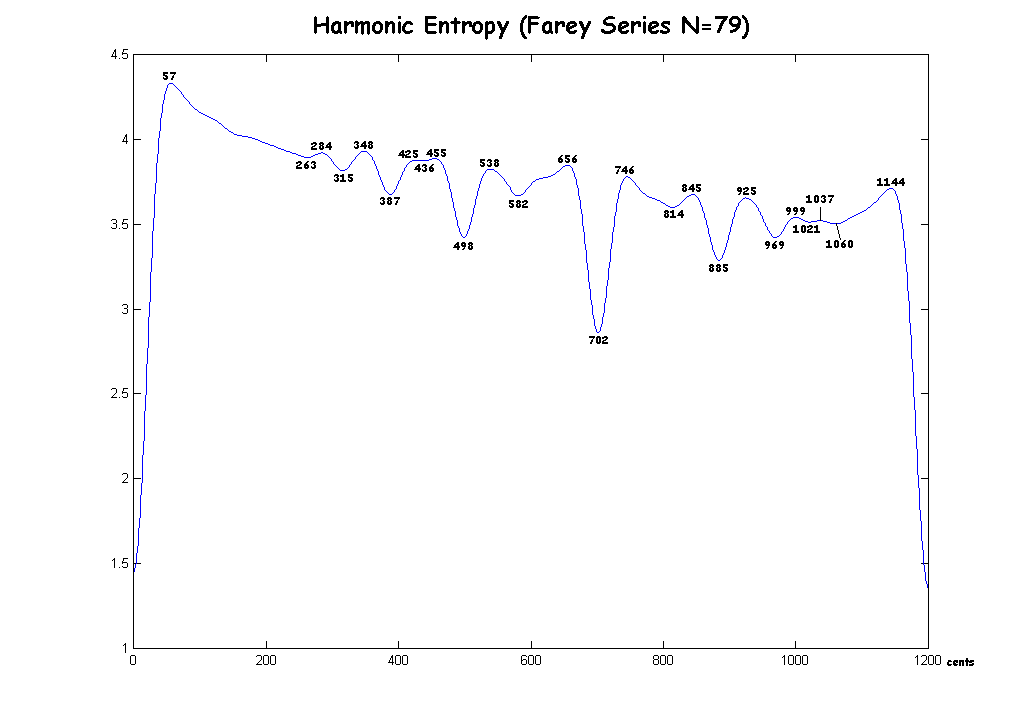Here is a graph for the Farey Series N=80: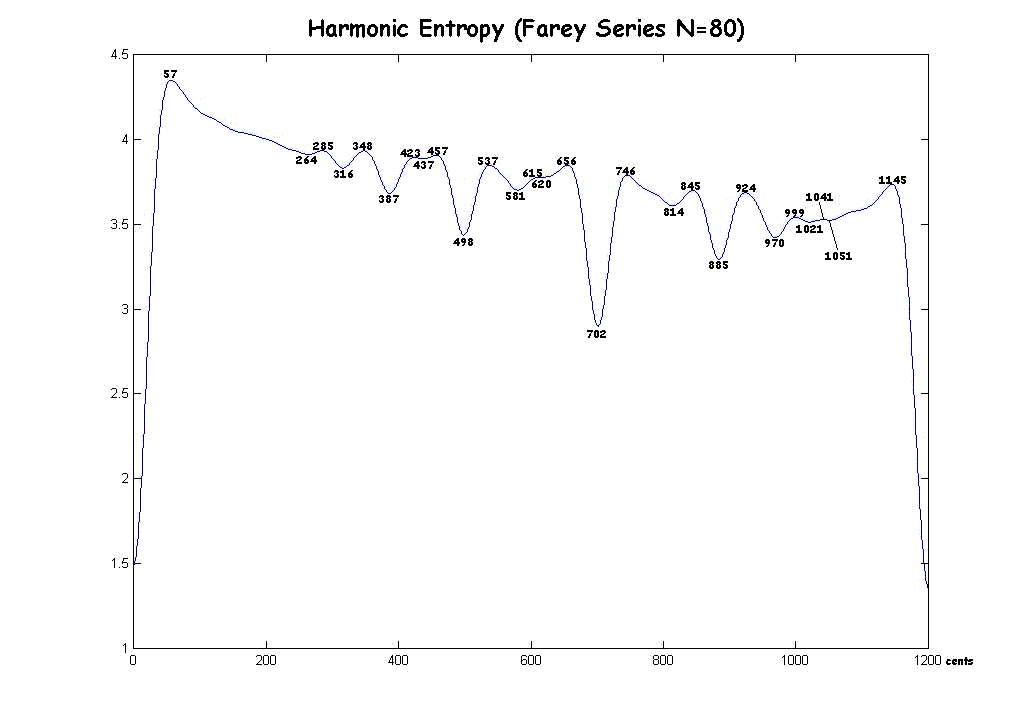Here is a graph for the Farey Series N=81: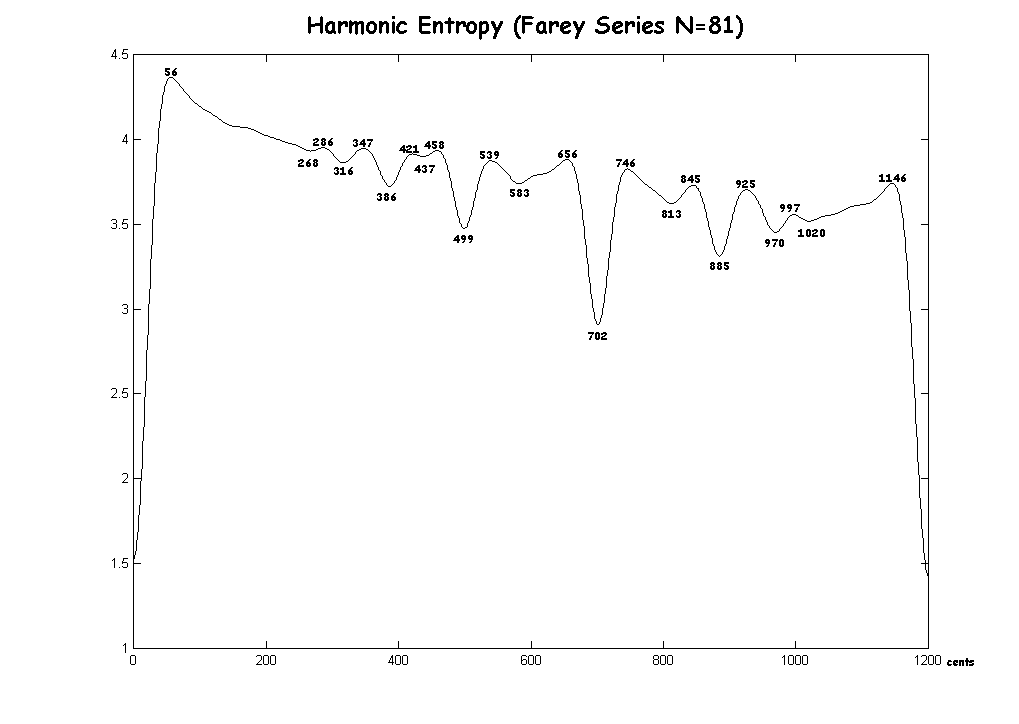Here is a graph for the six consecutive Farey Series N=79 to 84: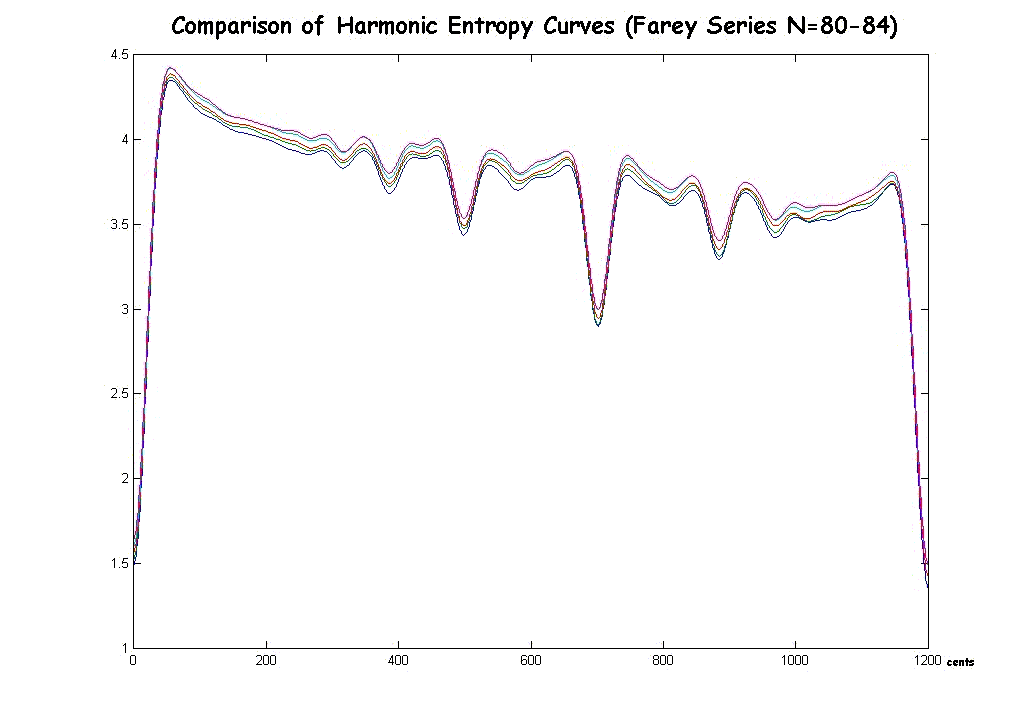Here is a graph for the Mann Series N=112, with the extrema labelled: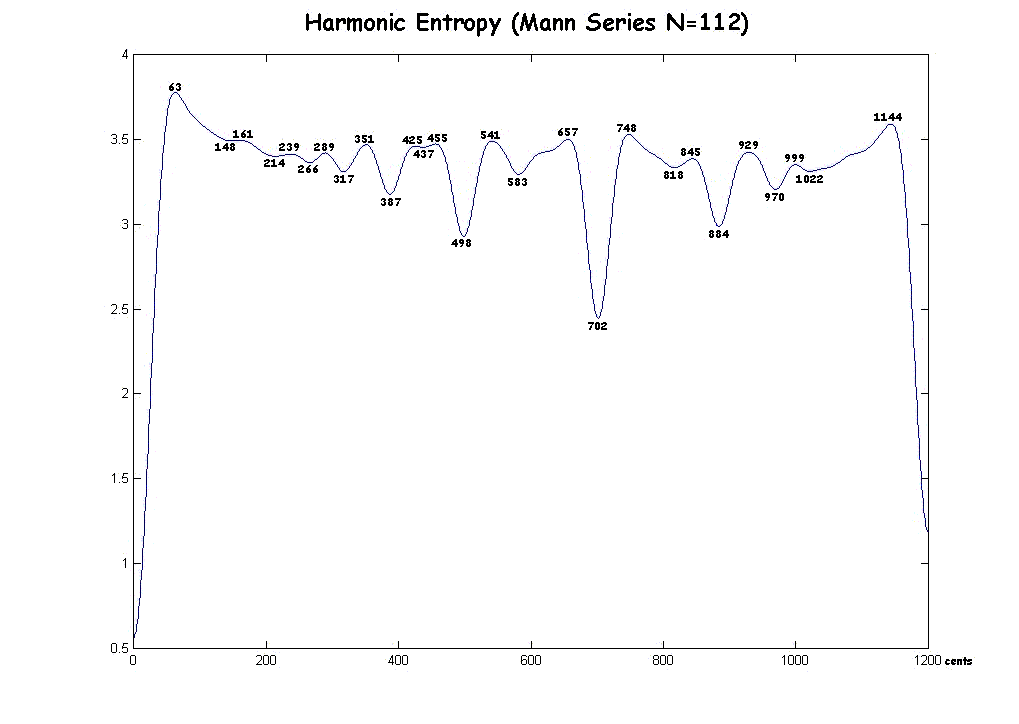Comparison of Mann Series entropy curves as N increases: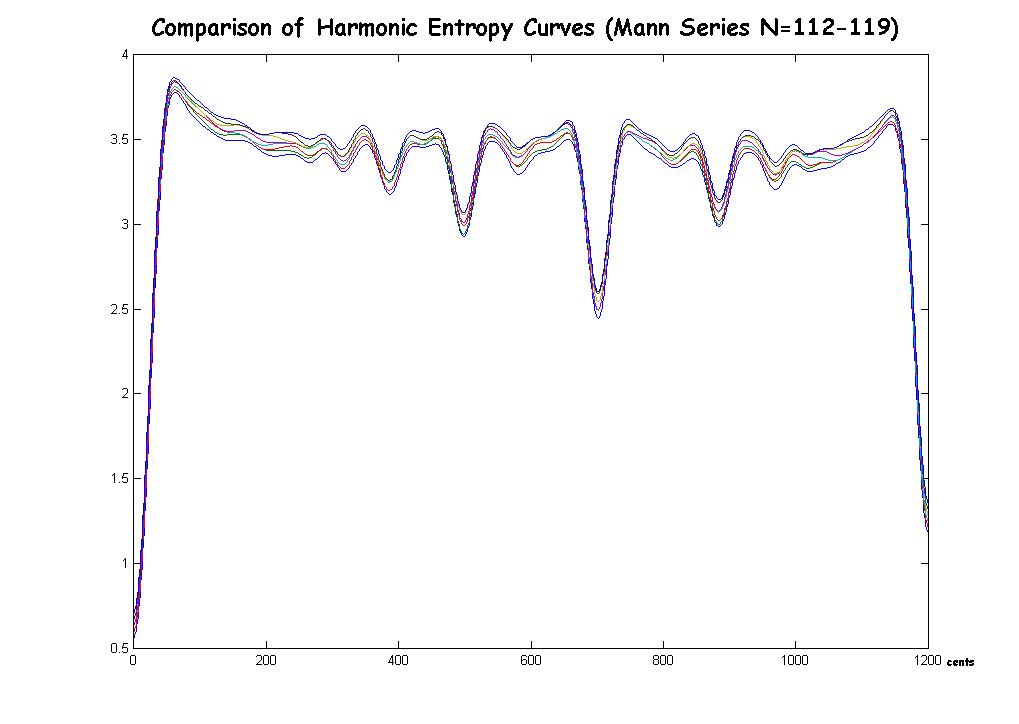The most important thing I left out was that local maxima and minima have limited relevance unless your music uses continuous sweeps of the interval spectrum. I have always held this as a (very mild) criticism of some of Sethares's arguments. It only takes a tiny change in the harmonic entropy function (say, a change of 1 in N) to convert a local maximum into a local minimum or vice versa. The value of the function need change only very little at any given interval, but the just ratios will tend to be near these local extrema. The values of the function are more important, however these are dependent on whether the allowed fractions in the analysis are defined to have numerator less than N, denominator less than N, numerator + denominator < 2N, etc. The choice of one of these rules is a difficult one, but the local extrema, I think, should be independent of this choice, which is why I only reported those.

Essentially, Erlich is asking what are the most complex possible ratios which can be consonant according to the periodicity criteria, for a "typical" listener (uncertainty of 1%).

If a larger uncertainty is used (i.e., a different pitch range) there will be fewer minima - i.e., more complex intervals will not have a minima near them.

If the higher note remains the same and the lower note is changed, numerator less than N, denominator always less than numerator, leads to a Farey series.

If the lower note remains the same and the higher note is changed, denominator less than N, numerator can go up arbitrarily high (to infinity).

If the center frequency remains the same, and both notes change,
numerator + denominator < 2N. (see Mann, An Analytical Study of Harmonic Intervals)

A note relating harmonic entropy to my concept of finity: more complex ratios tend to be understood as simpler ones.

Many thanks to Paul Erlich for giving permission to add to his posting, and to Dave Keenan for hosting the webpage while I didn't have FTP access.

. . . . . . . . .

Please help keep Tonalsoft online! Select your level of support from the menu. Thank you!

 support level: patron: \$50 USD savior: \$100 USD angel of tuning: \$500 USD microtonal god: \$1000 USD friend: \$25 USD donor: \$5 USD